Home > CC4 > Chapter A > Lesson A.1.8 > ProblemA-85

A-85.

Solve the equations below and check your solutions. Homework Help ✎

1. −3 + 2x = −x + 6

2. 5 − 3x = x + 1

3. −2x = 4x + 9

4. 4x + 3 = x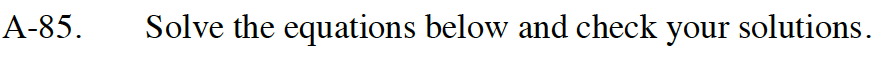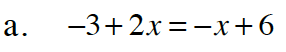Add 3 to both sides.

−3 + 2x = −x + 6
+3 +3
2x = −x + 9

Add x to both sides.

2x = −x + 9
+x +x
3x = 9

Divide both sides by 3.

x = 3

Substitute 3 back into the original equation.
− 3 + 2(3) = −(3) + 6
−3 + 6 = −3 + 6
−3 = −3

It works!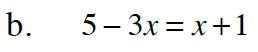Refer to part (a).

x = 1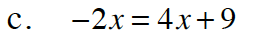x = −1.5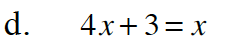Use the same method as parts (a), (b), and (c). Make sure to check your answer as shown in part (a).